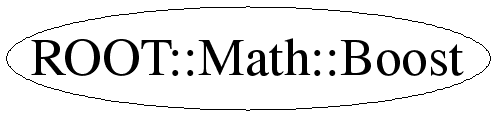# class ROOT::Math::Boost

```
Lorentz boost class with the (4D) transformation represented internally
by a 4x4 orthosymplectic matrix.
specialized Lorentz boosts.
Also, the 3-D rotation classes can be considered to be special Lorentz
transformations which do not mix space and time components.

@ingroup GenVector

```

## Function Members (Methods)

public:
 ~Boost() ROOT::Math::Boost::XYZVector BetaVector() const ROOT::Math::Boost Boost() ROOT::Math::Boost Boost(ROOT::Math::BoostX const& bx) ROOT::Math::Boost Boost(ROOT::Math::BoostY const& by) ROOT::Math::Boost Boost(ROOT::Math::BoostZ const& bz) ROOT::Math::Boost Boost(const ROOT::Math::Boost&) ROOT::Math::Boost Boost(double* begin, double* end) ROOT::Math::Boost Boost(ROOT::Math::Boost::Scalar beta_x, ROOT::Math::Boost::Scalar beta_y, ROOT::Math::Boost::Scalar beta_z) void GetComponents(double* begin) const void GetComponents(ROOT::Math::Boost::Scalar& beta_x, ROOT::Math::Boost::Scalar& beta_y, ROOT::Math::Boost::Scalar& beta_z) const void GetLorentzRotation(ROOT::Math::Boost::Scalar* r) const ROOT::Math::Boost Inverse() const void Invert() bool operator!=(const ROOT::Math::Boost& rhs) const ROOT::Math::LorentzVector > operator()(const ROOT::Math::LorentzVector >& v) const ROOT::Math::LorentzVector > operator*(const ROOT::Math::LorentzVector >& v) const ROOT::Math::Boost& operator=(ROOT::Math::BoostX const& bx) ROOT::Math::Boost& operator=(ROOT::Math::BoostY const& by) ROOT::Math::Boost& operator=(ROOT::Math::BoostZ const& bz) ROOT::Math::Boost& operator=(const ROOT::Math::Boost&) bool operator==(const ROOT::Math::Boost& rhs) const void Rectify() void SetComponents(const ROOT::Math::DisplacementVector3D,ROOT::Math::DefaultCoordinateSystemTag>& beta) void SetComponents(double* begin, double* end) void SetComponents(ROOT::Math::Boost::Scalar beta_x, ROOT::Math::Boost::Scalar beta_y, ROOT::Math::Boost::Scalar beta_z)
protected:
 void SetIdentity()

## Data Members

public:
 enum ELorentzRotationMatrixIndex { kLXX kLXY kLXZ kLXT kLYX kLYY kLYZ kLYT kLZX kLZY kLZZ kLZT kLTX kLTY kLTZ kLTT }; enum EBoostMatrixIndex { kXX kXY kXZ kXT kYY kYZ kYT kZZ kZT kTT };
private:
 ROOT::Math::Boost::Scalar fM

## Class Charts## Function documentation

Boost()
``` ========== Constructors and Assignment =====================

Default constructor (identity transformation)

```
`{ SetIdentity(); }`
Boost(ROOT::Math::Boost::Scalar beta_x, ROOT::Math::Boost::Scalar beta_y, ROOT::Math::Boost::Scalar beta_z)
```Construct given a three Scalars beta_x, beta_y, and beta_z

```
`{ SetComponents(beta_x, beta_y, beta_z); }`
Boost(const Avector & beta)
```Construct given a beta vector (which must have methods x(), y(), z())

```
`{ SetComponents(beta); }`
Boost(double* begin, double* end)
```Construct given a pair of pointers or iterators defining the
beginning and end of an array of three Scalars to use as beta_x, _y, and _z

```
`{ SetComponents(begin,end); }`
explicit Boost( BoostX const & bx )
```Construct from an axial boost

```
`{SetComponents(bx.BetaVector());}`
explicit Boost( BoostY const & by )
`{SetComponents(by.BetaVector());}`
explicit Boost( BoostZ const & bz )
`{SetComponents(bz.BetaVector());}`
operator=( BoostX const & bx )
``` The compiler-generated copy ctor, copy assignment, and dtor are OK.

Assign from an axial pure boost

```
`{ return operator=(Boost(bx)); }`
operator=( BoostY const & by )
`{ return operator=(Boost(by)); }`
operator=( BoostZ const & bz )
`{ return operator=(Boost(bz)); }`
void Rectify()
```Re-adjust components to eliminate small deviations from a perfect
orthosyplectic matrix.

```
SetComponents(ROOT::Math::Boost::Scalar beta_x, ROOT::Math::Boost::Scalar beta_y, ROOT::Math::Boost::Scalar beta_z)
``` ======== Components ==============

Set components from beta_x, beta_y, and beta_z

```
GetComponents(ROOT::Math::Boost::Scalar& beta_x, ROOT::Math::Boost::Scalar& beta_y, ROOT::Math::Boost::Scalar& beta_z) const
```Get components into beta_x, beta_y, and beta_z

```
SetComponents(const ROOT::Math::DisplacementVector3D<ROOT::Math::Cartesian3D<double>,ROOT::Math::DefaultCoordinateSystemTag>& beta)
```Set components from a beta vector

```
`{ SetComponents(beta.x(), beta.y(), beta.z()); }`
void SetComponents(double* begin, double* end)
```Set given a pair of pointers or iterators defining the beginning and end of
an array of three Scalars to use as beta_x,beta _y, and beta_z

```
void GetComponents(IT begin, IT end)
```Get given a pair of pointers or iterators defining the beginning and end of
an array of three Scalars into which to place beta_x, beta_y, and beta_z

```
XYZVector BetaVector()
GetLorentzRotation(ROOT::Math::Boost::Scalar* r) const
```Get elements of internal 4x4 symmetric representation, into a data
array suitable for direct use as the components of a LorentzRotation
Note -- 16 Scalars will be written into the array; if the array is not
that large, then this will lead to undefined behavior.

```
operator()(const ROOT::Math::LorentzVector<ROOT::Math::PxPyPzE4D<double> >& v) const
``` =========== operations ==============

Lorentz transformation operation on a Minkowski ('Cartesian')
LorentzVector

```
A4Vector operator*(const ROOT::Math::LorentzVector<ROOT::Math::PxPyPzE4D<double> >& v) const
```Overload operator * for boost on a vector

```
void Invert()
```Invert a Boost in place

```

```Return inverse of  a boost

```
return ! operator==(const ROOT::Math::Boost& rhs) const
void SetIdentity()

Last update: root/mathcore:\$Id: Boost.h 21468 2007-12-18 17:51:12Z moneta \$
Copyright (c) 2005 ROOT FNAL MathLib Team *

This page has been automatically generated. If you have any comments or suggestions about the page layout send a mail to ROOT support, or contact the developers with any questions or problems regarding ROOT.